How Cheenta works to ensure student success?
Explore the Back-Story

# Test of Mathematics Solution Subjective 113 - Vertices of a TriangleThis is a Test of Mathematics Solution Subjective 113 (from ISI Entrance). The book, Test of Mathematics at 10+2 Level is Published by East West Press. This problem book is indispensable for the preparation of I.S.I. B.Stat and B.Math Entrance.

## Problem:

Find the vertices of the two right angles triangles, each having area 18 and such that the point (2, 4) lies on the hypotenuse, and the other two sides are formed by the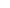and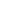axes.

## Solution:

Suppose the vertices are (a, 0) and (0, b). Clearly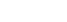or ab = 36.
Also the equation of the line through (0,a), (b,0) is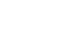. Since we know that (2, 4) is on that line, there fore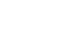.
In this equation, lets replace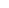by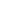. Hence we get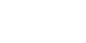or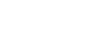.
Therefore we get a quadratic in b.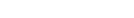. We can simply middle term factorize this to find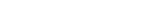. Thus b = 12 or 6 implying a = 3 or 6.
The other two vertices are: (0,12) and (3, 0) OR (0,6) and (6,0).

## Chatuspathi: Test of Mathematics Solution Subjective 113

• What is this topic: Coordinate Geometry
• What are some of the associated concept: Intercept form of straight line equation.
• Where can learn these topics:  I.S.I. & C.M.I. Entrance course of Cheenta, discusses these topics in the ‘Coordinate Geometry’ module.
• Book Suggestions: Coordinate Geometry Volume I by S.L. LoneyThis is a Test of Mathematics Solution Subjective 113 (from ISI Entrance). The book, Test of Mathematics at 10+2 Level is Published by East West Press. This problem book is indispensable for the preparation of I.S.I. B.Stat and B.Math Entrance.

## Problem:

Find the vertices of the two right angles triangles, each having area 18 and such that the point (2, 4) lies on the hypotenuse, and the other two sides are formed by theandaxes.

## Solution:

Suppose the vertices are (a, 0) and (0, b). Clearlyor ab = 36.
Also the equation of the line through (0,a), (b,0) is. Since we know that (2, 4) is on that line, there fore.
In this equation, lets replaceby. Hence we getor.
Therefore we get a quadratic in b.. We can simply middle term factorize this to find. Thus b = 12 or 6 implying a = 3 or 6.
The other two vertices are: (0,12) and (3, 0) OR (0,6) and (6,0).

## Chatuspathi: Test of Mathematics Solution Subjective 113

• What is this topic: Coordinate Geometry
• What are some of the associated concept: Intercept form of straight line equation.
• Where can learn these topics:  I.S.I. & C.M.I. Entrance course of Cheenta, discusses these topics in the ‘Coordinate Geometry’ module.
• Book Suggestions: Coordinate Geometry Volume I by S.L. Loney

### Knowledge Partner